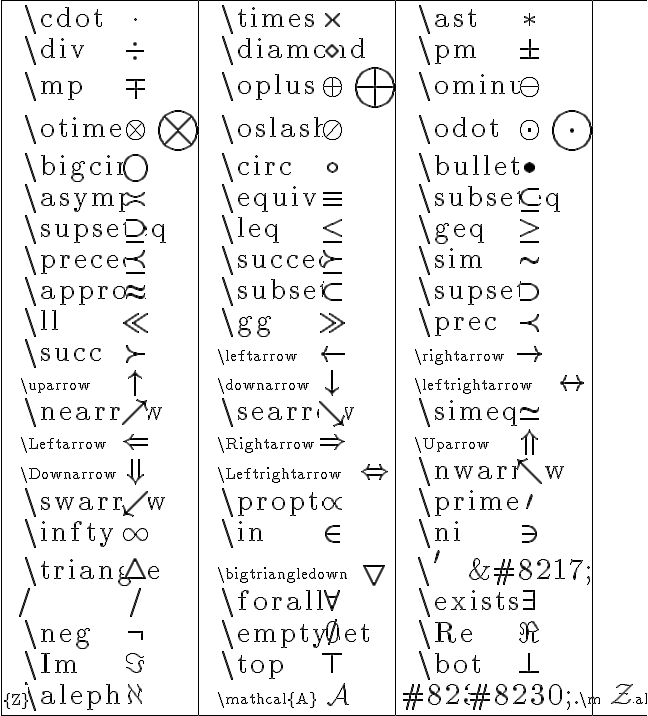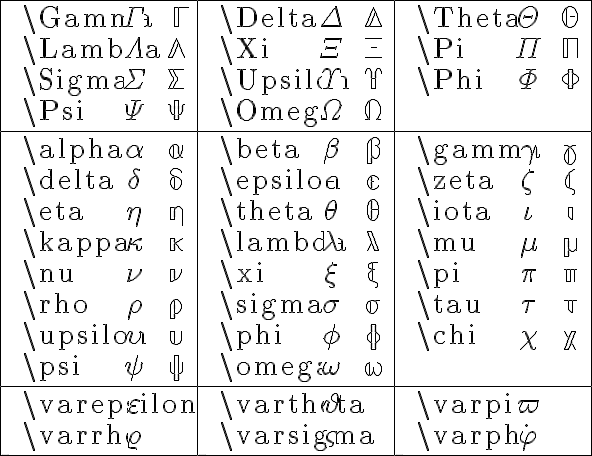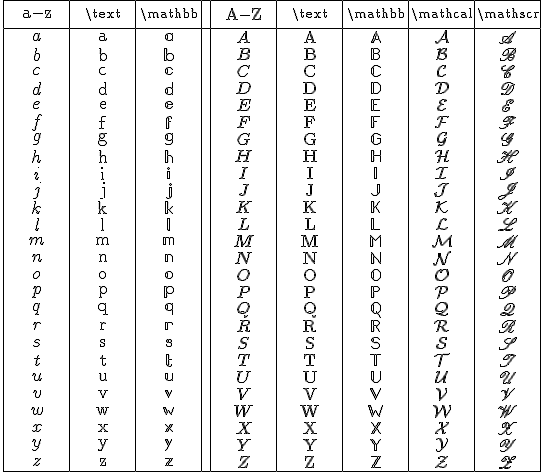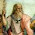## Wednesday, August 26, 2009

### Latex rendering update

Using equation on this blog asks that you put $sign at the beginning and$sign at the end to substitute in bracket [ ]-tex and / tex

Here is the site language that will help blog developers with their latex language and give them an alternative from having to shift over to word press.

Test

$\odot$ $\oplus$ $\pi$ $\omega$

$\LARGE U=\frac{-GMm}r=\frac{-GMh}{rc^2}{vo}$

$\large hv=hv_o[{1-}\frac{GM}{rc^2}] \hspace9 v=v_o[{1-}\frac{GM}{rc^2}] \hspace9 \frac{\bigtriangledown v} {v_o}={-}\frac{GM}{rc^2}$

***Click on Image for a larger sizeClick on Image for a larger sizeClick on Image for a larger size

***

See:Help:Latex Symbols-Mathematics

1.Testing comment section

$\LARGE U=\frac{-GMm}r=\frac{-GMh}{rc^2}{vo}$

2.For a post with lots of math symbols you probably need to remove the border on the image by fiddling around with the css.

3.Hi Watchmath,

Thanks for taking the time.

Under a blog format what would I look for? I posted it under the script you had given so I am not sure how this is translated through to the blog format? Any pointers or ideas here?

Best,

4.$2+6x*sqrt(9)$

5.Napoleon10:04 AM

Thanks for the post, I was searching for the \mathcal{X} symbol's command for a long time.